# The GLIMMIX Procedure

You can add a multiplicative overdispersion parameter to a generalized linear model in the GLIMMIX procedure with the statement

random _residual_;


For models in which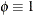, this effectively lifts the constraint of the parameter. In models that already contain aor k scale parameter—such as the normal, gamma, or negative binomial model—the statement adds a multiplicative scalar (the overdispersion parameter,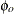) to the variance function.

The overdispersion parameter is estimated from Pearson’s statistic after all other parameters have been determined by (restricted) maximum likelihood or quasi-likelihood. This estimate is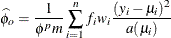where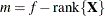if the NOREML option is in effect, and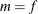otherwise, and f is the sum of the frequencies. The power p is –1 for the gamma distribution and 1 otherwise.

Adding an overdispersion parameter does not alter any of the other parameter estimates. It only changes the variance-covariance matrix of the estimates by a certain factor. If overdispersion arises from correlations among the observations, then you should investigate more complex random-effects structures.